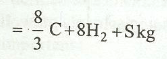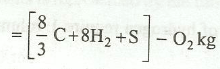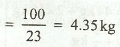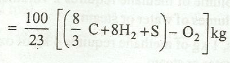Theoretical or Minimum Air Required for Complete Combustion

The theoretical or minimum mass (or volume) of oxygen required for complete combustion of 1 kg of fuel may be calculated from the chemical analysis of the fuel. In order to obtain maximum amount of heat from a fuel, the adequate supply of oxygen is very essential for the complete combustion of a fuel.

The mass of oxygen, required by each of the constituents of the fuel, may be calculated from the chemical equations given in previous topic about "combustion of fuels". Now consider 1 kg of a fuel.

We know that 1 kg of carbon requires 8 / 3 kg of oxygen for its complete combustion. Similarly, 1 kg of hydrogen requires 8 kg of oxygen and 1 kg of sulphur requires 1 kg of oxygen for its complete combustion.

Total oxygen required for complete combustion of 1 kg of fuel is given as:If some oxygen (say O2 kg) is already present in the fuel, then total oxygen required for the complete combustion of 1 kg of fuel is:It may be noted that the oxygen has to be obtained from the atmospheric air, which mainly consists of nitrogen and oxygen.

The composition of air is taken as:

Nitrogen (N2) = 77% ; Oxygen (O2) = 23%  (By Mass)

and

Nitrogen (N2) = 79%; Oxygen (O2) = 21%  (By Volume)

It is thus obvious, that for obtaining 1 kg of oxygen, amount of air required:Theoretical or minimum air required for complete combustion of 1 kg of fuel:1.